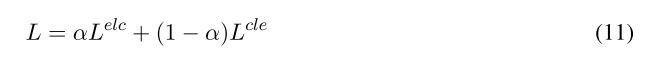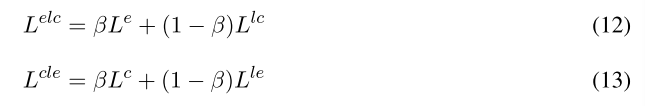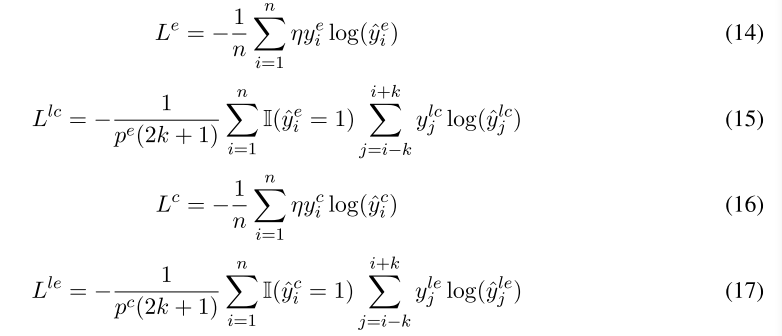2020COLING-《A Symmetric Local Search Network for Emotion-Cause Pair Extraction》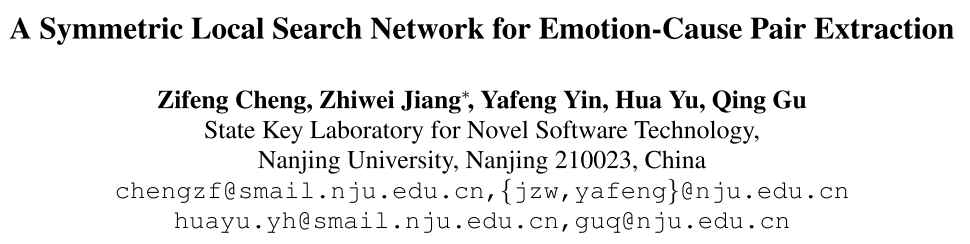# 1. 摘要和介绍

SLSN包含两个对称子网络，名为情感子网络和原因子网络，每一个子网络都包含一个子句表示学习器和一个局部对搜索器（local pair searcher, LPS）。

• 提出了对称局部搜索网络模型，这是一个端到端的模型，是解决ECPE问题的新方式。
• 在SLSN中设计了LPS，它能够同时对情感和原因子句进行检测和匹配。
• 实验证明了SLSN的有效性。

# 2. 对称局部搜索网络

## 2.2 SLSN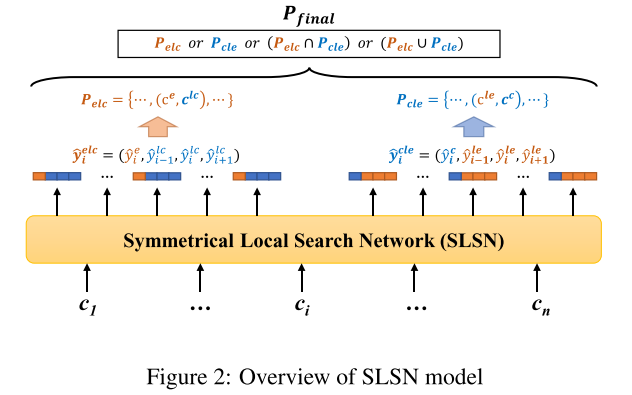E-LC标签$\hat{y}_i^{elc}$包含第$i$个子句的情感标签$\hat y_i^e$和局部原因标签($\hat{y}_{i-1}^{lc}, \hat{y}_{i}^{lc}, \hat{y}_{i+1}^{lc}$)

C-LE标签$\hat{y}_i^{cle}$包含第$i$个子句的原因标签$\hat y_i^c$和局部情感标签($\hat{y}_{i-1}^{le}, \hat{y}_{i}^{le}, \hat{y}_{i+1}^{le}$)

$\hat{y}_i^{elc}=(1,1,0,0)$，意味着$(c_i,c_{i-1})$是情感-原因子句，其余不是；

$\hat{y}_i^{cle}=(1,1,0,0)$，意味着$(c_{i-1}, c_i)$是情感-原因子句，其余不是。

SLSN的两种局部搜索器会得到两个情感-原因对集合，我们可以对这两个集合取交集、并集等，得到最终的情感-原因对。

## 2.3 SLSN的组件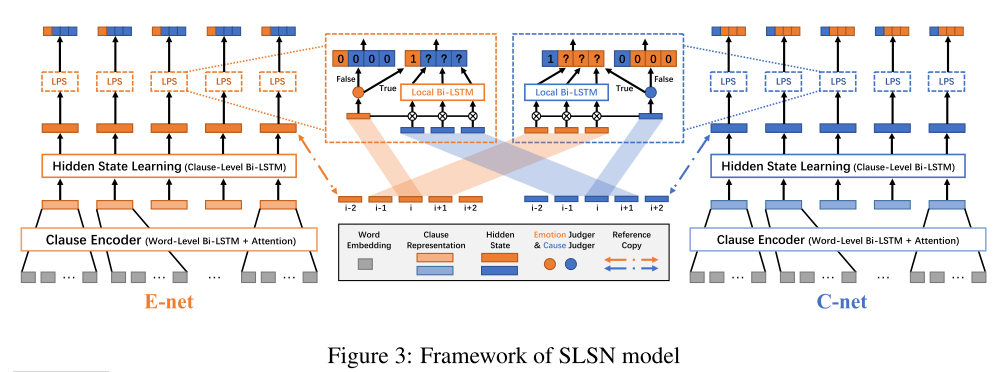### 2.3.2 子句编码器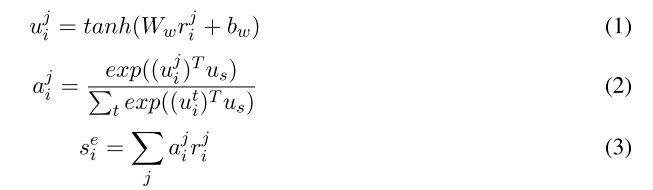### 2.3.4 局部搜索器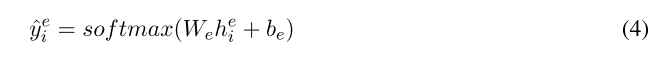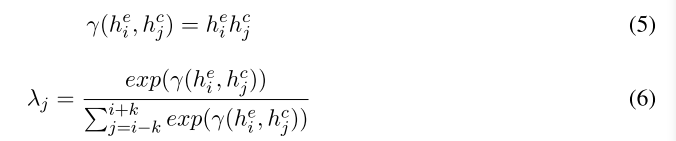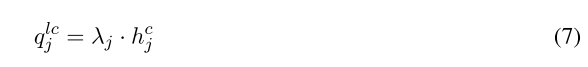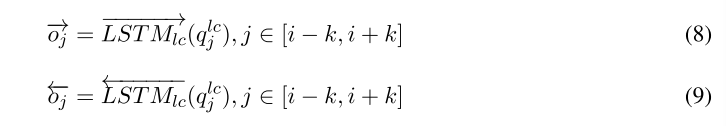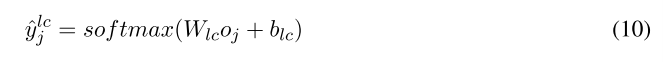C-LE过程与之类似，不再赘述。

## 2.4 模型训练